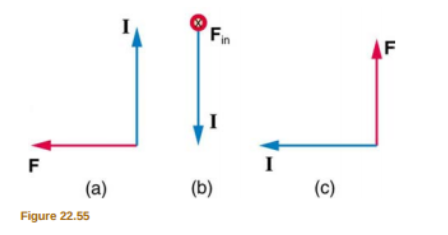# Problem: What is the direction of the magnetic field that produces the magnetic force shown on the currents in each of the three cases in Figure 22.55, assuming B is perpendicular to I?

###### FREE Expert Solution

In this problem, we're going to use the right-hand rule to determine the direction of the magnetic field.

84% (463 ratings)###### Problem Details

What is the direction of the magnetic field that produces the magnetic force shown on the currents in each of the three cases in Figure 22.55, assuming B is perpendicular to I?Frequently Asked Questions

What scientific concept do you need to know in order to solve this problem?

Our tutors have indicated that to solve this problem you will need to apply the Magnetic Force on Current-Carrying Wire concept. You can view video lessons to learn Magnetic Force on Current-Carrying Wire. Or if you need more Magnetic Force on Current-Carrying Wire practice, you can also practice Magnetic Force on Current-Carrying Wire practice problems.

What professor is this problem relevant for?

Based on our data, we think this problem is relevant for Professor Maune's class at GSU.